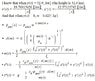# Modelling the motion of a meteor

• PeterH
In summary, the conversation is about modeling the motion of a meteor through the atmosphere, taking into account factors such as air resistance, air density, and mass loss upon impact. The equations used and the need for numerical solution are discussed, with a suggestion to calculate it step by step. It is mentioned that there are better integration schemes and the use of a numerical solver is preferred. Some confusion arises over the equations and variables involved, with a request for clarification and a suggestion to simplify one of the equations. The topic of atmospheric density is also briefly mentioned.

#### PeterH

Hi!
I am to model the motion of a meteor as it travels through the atmosphere, taking into account the loss of mass, which is 0.025 kg upon impact (height = 0). I also have to take into account the air resistance on the meteor, the fact that the air density is a function of height and that the orthographic projection of the meteor perpendicular the direction of movement (part of the air resistance equation) is a function of the mass of the meteor.
I assume that gravitational field strength is constant.

I have attached a picture of the equations I have been able to derive so far using standard formulas.

I have to solve the differential equations for x(t) and y(t) numerically, however, I fail to see how and therefore seek help.

This is for a very important school project and I'm running out of time, so I really need help. Even the smallest hint would be greatly appreciated!

#### Attachments

•Mat.jpg
40.6 KB · Views: 436
Simply calculate it step by step in small time steps?
You know the initial position and velocity at t=0, you can calculate the acceleration there and use this to estimate the position and velocity at t=0.01s (or whatever). Use the data there to estimate the values for t=0.02s and so on. There are better integration schemes, but start with the easiest one and improve that later if you like.

Your equation for A can be simplified a bit.

The atmospheric density does not follow an exponential law as the temperature depends on height, by the way.

Newtonian gravity with atmospheric drag is not solvable analytically. Use an ordinary differential equation numerical solver. I prefer MATLAB.

It would help if you tell us what exactly A(m), m(t), etc are. Also, what is that little squiggly variable for? Is it a constant?

Last edited:
I don't think that equation for m(t) makes sense, it has m(t) at the right side as well, but multiplied by t and other factors. Looks like a wrong differential equation.